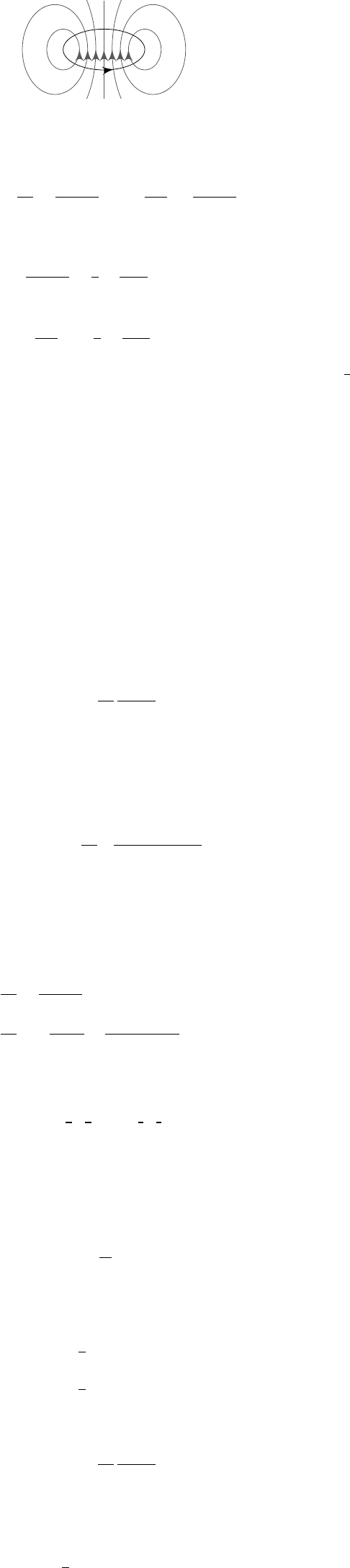3Magnetostatics

IB Electromagnetism3.3 Magnetic dipoles
According to Maxwell’s equations, magnetic monopoles don’t exist. However, it
turns out that a localized current looks like a dipole from far far away.
Example
(Current loop)
.
Take a current loop of wire
C
R
and current
I.
Based on the fields generated by a straight wire, we can guess that
B
looks like
this, but we want to calculate it.
By the Biot-Savart law, we know that
A(r) =
µ
0
4π
Z
J(r
0
)
|r r
0
|
dV =
µ
0
I
4π
I
C
dr
0
|r r
0
|
.
Far from the loop, |r r
0
| is small and we can use the Taylor expansion.
1
|r r
0
|
=
1
r
+
r · r
0
r
3
+ ···
Then
A(r) =
µ
0
I
4π
I
C
1
r
+
r · r
0
r
3
+ ···
dr
0
.
Note that
r
is a constant of the integral, and we can take it out. The first
1
r
term vanishes because it is a constant, and when we integrate along a closed
loop, we get 0. So we only consider the second term.
We claim that for any constant vector g,
I
C
g · r
0
dr
0
= S × g,
where
S
=
R
d
S
is the vector area of the surface bounded by
C
. It follows from
Green’s theorem for the function f(r
0
):
I
C
f(r
0
)dr
0
=
Z
S
f × dS,
taking f (r
0
) = g · r
0
. Then
I
C
g · r
0
dr
0
=
Z
S
g × dS = S ×g.
Using this, we have
A(r)
µ
0
4π
m × r
r
3
,
where
Definition (Magnetic dipole moment). The magnetic dipole moment is
m = IS.
Then
B = × A =
µ
0
4π
3(m ·
ˆ
r)
ˆ
r m
r
3
.
This is the same form as
E
for an electric dipole, except that there is no 1
/r
2
leading term here, because we have no magnetic monopoles.
After doing it for a loop, we can do it for a general current distribution:
Example. We have
A
i
(r) =
µ
0
4π
Z
J
i
(r
0
)
|r r
0
|
dV
0
=
µ
0
4π
Z
J
i
(r
0
)
r
J
i
(r
0
)(r · r
0
)
r
3
+ ···
dV
0
.
We will show that the first term vanishes by showing that it is a total derivative.
We have
0
j
(J
j
r
0
i
) = (
0
j
J
j
)
| {z }
=∇·J=0
r
0
i
+ (
0
j
r
0
i
)
|{z}
=δ
ij
J
j
= J
i
.
For the second term, we look at
0
j
(J
j
r
0
i
r
0
k
) = (
0
j
J
j
)r
0
i
r
0
k
+ J
i
r
0
k
+ J
k
r
0
i
= J
k
r
0
i
+ J
i
r
0
k
.
Apply this trick to
Z
J
i
r
j
r
0
j
dV =
Z
r
j
2
(J
i
r
0
j
J
j
r
0
i
) dV
0
,
where we discarded a total derivative
0
j
(
J
j
r
0
i
r
0
u
). Putting it back in vector
notation,
Z
J
i
r · r
0
dV =
Z
1
2
(J
i
(r · r
0
) r
i
(J · r
0
)) dV
=
Z
1
2
[r × (J × r
0
)]
i
dV.
So the long-distance vector potential is again
A(r) =
µ
0
4π
m × r
r
3
,
with
Definition (Magnetic dipole moment).
m =
1
2
Z
r
0
× J(r
0
) dV
0
.